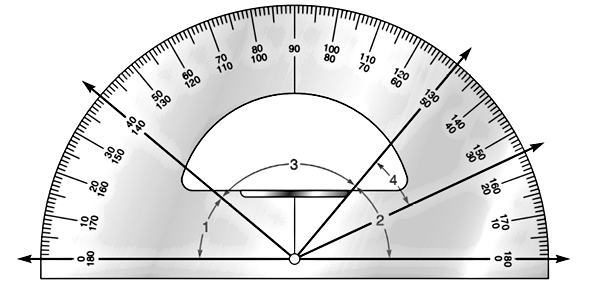Chapter 1.2, Problem 20E### Elementary Geometry for College St...

6th Edition
Daniel C. Alexander + 1 other
ISBN: 9781285195698

#### Solutions

Chapter
Section### Elementary Geometry for College St...

6th Edition
Daniel C. Alexander + 1 other
ISBN: 9781285195698
Textbook Problem
1 views

# Using the drawing for Exercise 19, estimate the measure of each angle to the nearestmultiple of 5 ° (such as, 20 ° , 25 ° , 30 ° , etc.). a) m ∠ 2 b) m ∠ 4To determine

a) To estimate:

The measure of the angle m2 to the nearest multiple of 5°.

Explanation

Calculation:

Given,

Thus,

In the above given drawing the measure of the m2 is measured from the inner scale, since; one of the side of the angle reads Zero at the inner scale

To determine

The measure of the angle m4 to the nearest multiple of 5°.

### Still sussing out bartleby?

Check out a sample textbook solution.

See a sample solution

#### The Solution to Your Study Problems

Bartleby provides explanations to thousands of textbook problems written by our experts, many with advanced degrees!

Get Started

#### find the real roots of each equation by factoring. 132. 6x2 + x + 12 = 0

Applied Calculus for the Managerial, Life, and Social Sciences: A Brief Approach

#### Find the domain of the function. 36. f(u)=u+11+1u+1

Single Variable Calculus: Early Transcendentals, Volume I

#### Evaluate .

Study Guide for Stewart's Single Variable Calculus: Early Transcendentals, 8th